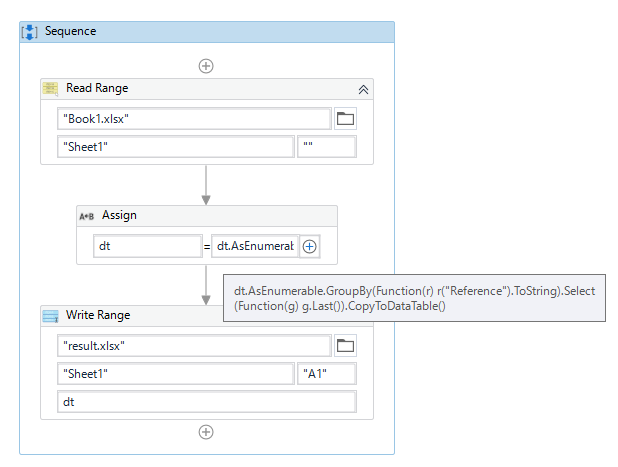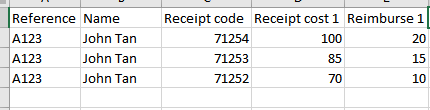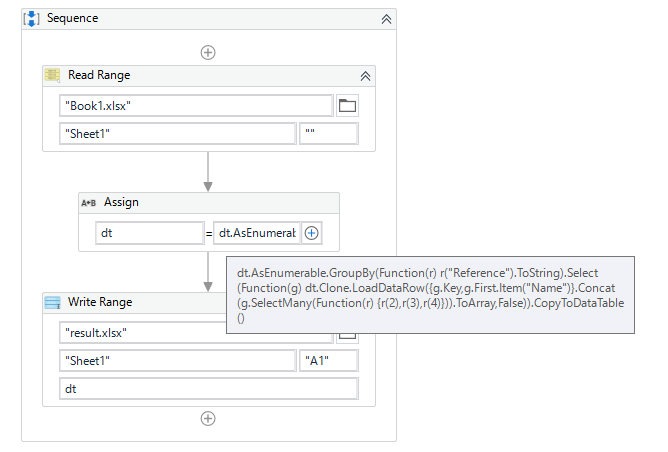# Uipath Excel to check if value is in column, if so enter value in a new column

Hello all,

Would like to seek your help for this problem of mine.

I’m trying to read through a column and if a value of the column is a duplicate in the column, input the row values in the original.

In this case, the reference(A2 & A3) is the same, so row A3 should be deleted and C3,D3 and E3 will be transferred to F2,G2 and H2 instead.

I’ve use Excel Application Scope, read range and for each row and then im stuck,

Hi,

How about the following sample? Does this work for you?``````dt = dt.AsEnumerable.GroupBy(Function(r) r("Reference").ToString).Select(Function(g) g.Last()).CopyToDataTable()
``````

Sample20220124-1.zip (9.4 KB)

Regards,

Hello Yoichi,

It dont quite work as I want the end result to be like this instead.

And if the rows increase,It will result in this,

Do let me know if you’ve found a solution.

Hi,

I just understood your requirement. Can you try the following sample?``````dt = dt.AsEnumerable.GroupBy(Function(r) r("Reference").ToString).Select(Function(g) dt.Clone.LoadDataRow({g.Key,g.First.Item("Name")}.Concat(g.SelectMany(Function(r) {r(2),r(3),r(4)})).ToArray,False)).CopyToDataTable()
``````

Sample20220124-1v2.zip (9.7 KB)

Regards,

This topic was automatically closed 3 days after the last reply. New replies are no longer allowed.